Calculate Average in Microsoft Excel  Step by Step Guide ▷ 2020

While it is true, Excel is a program that is part of the renowned Microsoft Office suite and consists of spreadsheet software that, generally, used to carry out math and / or financial tasks based on any setting (academic, professional, business, etc.).

In this sense, it offers the possibility of perform all kinds of numerical calculations to manage any data flow effectively. For this reason, it allows simple mathematical operations and among these, is calculate the average of a set of numbers, easily and quickly.

Therefore, if you need generate reports in which you must present the mean of in a certain data set, it is recommended that you use Excel for this. So, in order for you to perform the necessary procedure correctly, in this post we explain how to obtain such numerical information through this program.

What is an average or average in Microsoft Excel and when is it necessary to obtain it?

In mathematics and statistics, a mean or average also known as “The arithmetic mean”, refers to the result that is obtained when performing a division with the sum of various quantities by the digit that represents them in their entirety, basically. That is, is the characteristic value of a series of quantitative data.

In other words, it is a number that results from the study or the sum of a set of values by dividing it by the number of addends. Therefore, it consists of a finite value that, in order to obtain it, it will be necessary to have at least two related quantities, in order to discover its midpoint.

Now, thanks to its usefulness for different studies, the mean has been classified as a digit of great interest, due to the fact that be able to measure the central tendency or the location of the center of a group of numbers within a statistical distribution.

Thus, it will be necessary to calculate an arithmetic mean in the following cases:

• In education, it is usually used to obtain the indicator of the academic level of a student.
• It also allows to verify the average value of the profits that a company acquires for one year or at any time interval.
• Is used to know the average of the days that a certain employee worked and those that did not.
• In environmental terms, it is useful for measure the central tendency of temperature for a day or a week, for example.
• It is a very juicy digit for conducting research based on economics. For example, the average inflation rates.

For its part, to better understand the concept of an average, it is worth introducing a small sample of how this numeric value works below:

• First of all, you have a group of digits that are: 2, 4, 6, 8, 10 and 12.
• So, once you do the summation of this set of numbers, you can get a total of 42.
• Now, it is verified that 6 numbers are part of said set. Well, all you need to do is divide the sum by the total number of digits there are, which would be: 42 in 6, in order to obtain an average of 7.

Steps to get the mean of a data set in Microsoft Excel

However, despite the fact that it is a very simple numerical operation when there are few numerical data to relate, the truth is that this is noticeably complicated by having to calculate the average between a much larger set of digits. Therefore, Microsoft Excel has designed a function that allows you to do this automatically.

In this sense, to enjoy remarkable simplicity when managing your numerical data and be able to calculate the mean of said set of values, it is important that you know how to carry out the procedure from Excel, in a timely manner and here, we mention each step to follow:

• To compute the arithmetic mean of several numbers found in a contiguous row or column in an Excel sheet, you must start with access the program document where the data to be studied are.
• After that, you have to click in a cell below or to the right of the numbers (which will depend on how they are arranged, either horizontally in rows or vertically in columns). In this case, we will have to click on a cell below.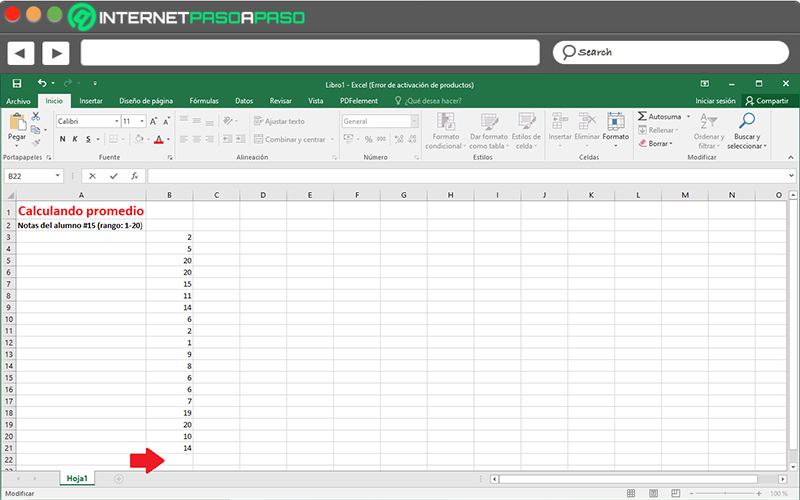• Next, it’s time to select the tab “Home” from the ribbon, then click on the arrow located on the option “Autosum” (located in the Modify group) and within the options displayed, just click on “Average”.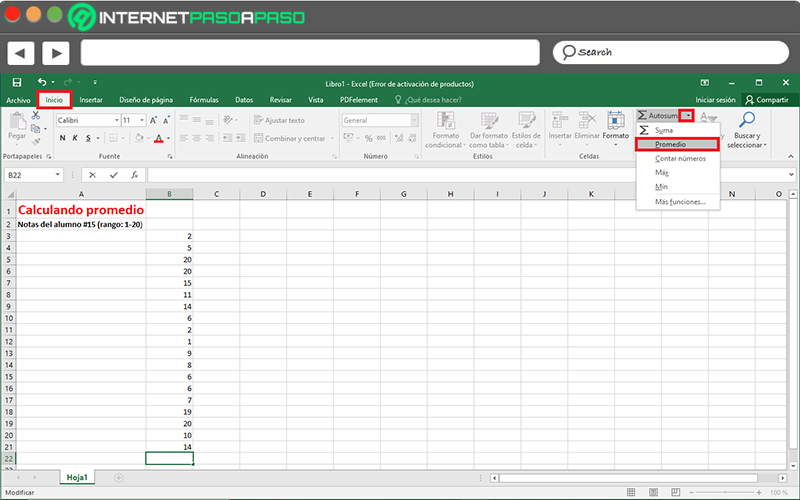• Finally, automatically, the entire row or column where the digits to be calculated are expressed will be selected and at that moment, you have to press the Enter key.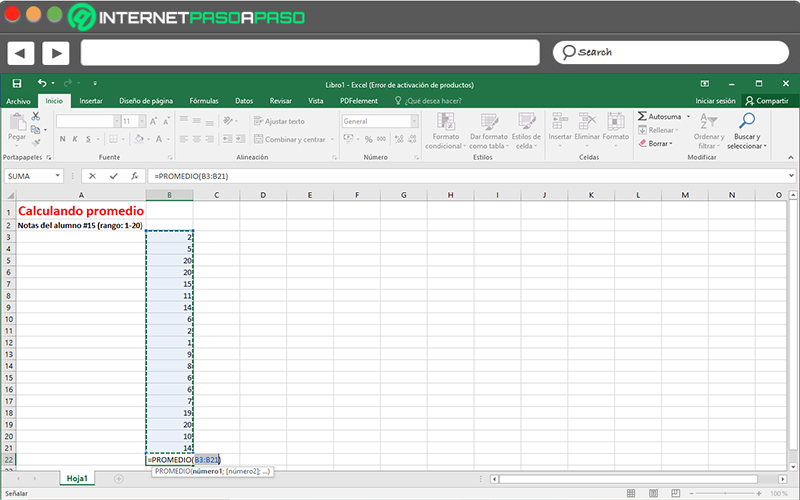• Done the above, right in the cell you have chosen at the beginning, you will get the central value or the arithmetic mean.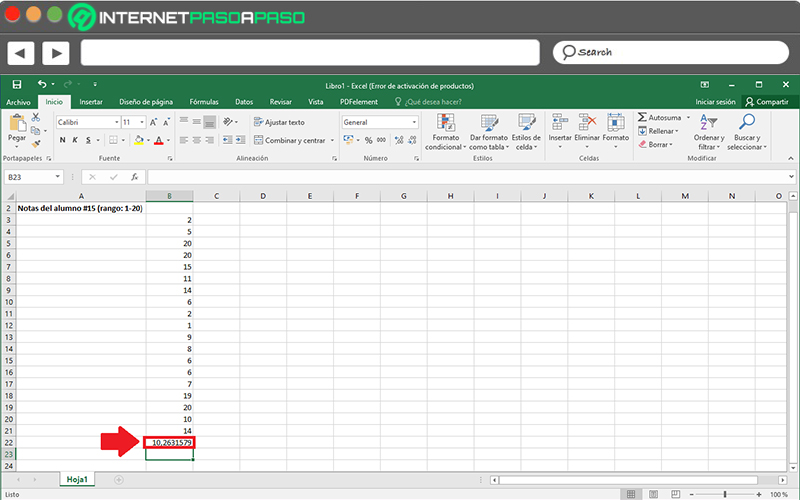In addition to this mechanism to follow, also there is another more practical way to calculate the mean of a set of numerical values making use of Microsoft Excel. Next, we explain what it consists of:

• First of all, find and enter the spreadsheet document where all the data to be averaged are found.
• Followed by that, select the entire row or column of those digits and click on the box shown in the lower right corner of the last cell (in this case because it is vertical).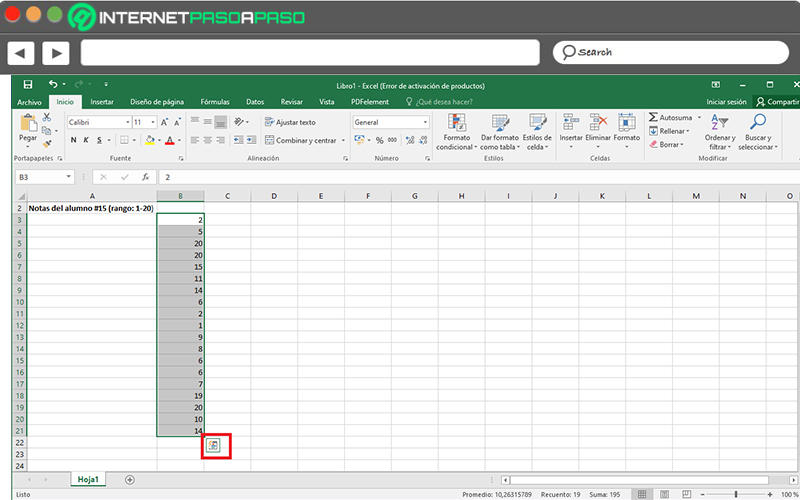• Now, among all the options shown below, click on the “Totals” tab and there, press where it says “Average”. Immediately, that value will be displayed in the next cell.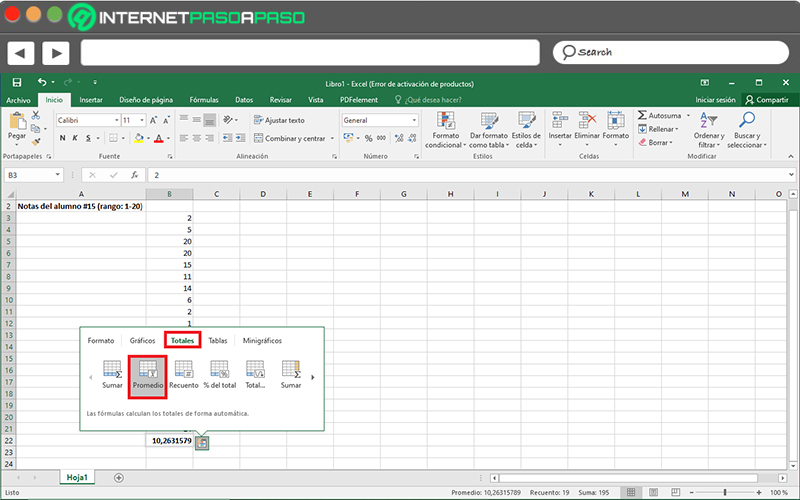As you will see, this last procedure is a way much faster to obtain and / or calculate the arithmetic mean of any data set displayed in a Microsoft Excel sheet. Taking into account that, whatever route you take for it, the result will be the same using this program.

If you have any questions, leave them in the comments, we will answer you as soon as possible, and it will surely be of great help to more members of the community. Thank you! 😉

Rate this post